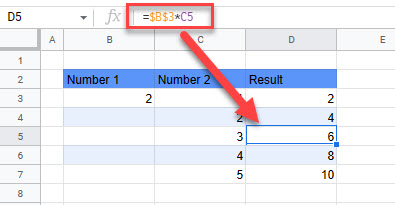# Keep Variable Cell Constant in Excel & Google Sheets

This tutorial demonstrates how to keep variable cells constant in Excel and Google Sheets.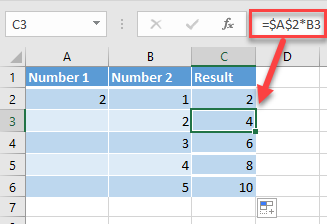## Keep Cells Constant in Formulas

When you copy a formula, cell references in the formula change depending on where you paste. If you want to keep variable cells constant, you can lock them before copying the formula. In this example, Column C is the result of the multiplication of values from A2 (2) and Column B.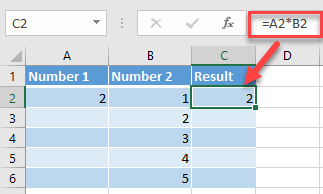If you copy the formula down Column C, both cell references change (A2 to A3 and B2 to B3, etc.).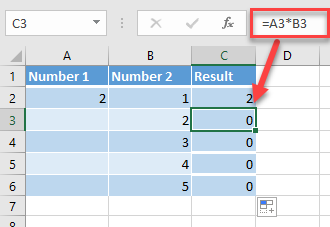Say you want to keep cell A2 constant. To do that, click on the cell reference in the formula bar (A2), and enter \$ before the column and row (\$A\$2).

You can also press F4 while in the formula bar to add the same dollar signs (before column reference and row reference). If you continue to press F4, it cycles through more absolute/relative referencing options.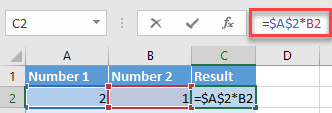As a result, Column C’s formula uses cell A2 as a constant, and the values from Column B are multiplied by 2 in every row.Note: In the newer version of Excel, there are new arrays formulas that automatically spill the values to the range, and don’t need any cell locking. These formulas include SORT, UNIQUE, SORTBY, and XLOOKUP.

## Keep Variable Cells Constant in Google Sheets

You can use the same logic to make variable cells constant in Google Sheets.